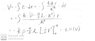# Potential difference between the center of a sphere and a point 4.0 cm away?

hidemi
Homework Statement:
A nonconducting sphere of radius 10 cm is charged uniformly with a density of 100 nC/m^3. What is the magnitude of the potential difference between the center and a point 4.0 cm away?

Relevant Equations:
V = kQ / r
I can use integral as attached to obtain the answer.
However, I wonder why I can not use " V = KQ / r " the get the correct answer.
Thanks.

#### Attachments

•1.jpg
23.3 KB · Views: 125

Homework Helper
Gold Member
2022 Award
However, I wonder why I can not use " V = KQ / r " the get the correct answer.
Thanks.
How exactly did you use that? E.g. what value for Q?

hidemi
The Q value I plug in is density*4pi/3*r^3, so V = k (density*4pi/3*r^2)
My confusion is that instead of using V = kQ/r directly, we integrate electric field (E) to calculate the potential (V) to obtain the correct answer?

Homework Helper
Gold Member
2022 Award
The Q value I plug in is density*4pi/3*r^3, so V = k (density*4pi/3*r^2)
My confusion is that instead of using V = kQ/r directly, we integrate electric field (E) to calculate the potential (V) to obtain the correct answer?
kQ/r would be the potential at a point P at radius r, ignoring any charges outside radius r, and taking the potential at infinity to be zero. This is not the same as finding the potential difference between P and the origin, O.
There is a potential due to the charges beyond radius r, but that is the same for both P and O. The difference is that the sphere within radius r creates a potential at O. Since you want the difference in potentials, this needs to be subtracted from kQ/r.

hidemi
kQ/r would be the potential at a point P at radius r, ignoring any charges outside radius r, and taking the potential at infinity to be zero. This is not the same as finding the potential difference between P and the origin, O.
There is a potential due to the charges beyond radius r, but that is the same for both P and O. The difference is that the sphere within radius r creates a potential at O. Since you want the difference in potentials, this needs to be subtracted from kQ/r.
I know V at origin is 0 for nonconducting sphere. I just wonder why can't we plug in 0.04m directly into the equation to get the potential difference, instead we need to integrate the electric field from 0-0.04m to calculate the potential?

Gordianus
$$V(B)-V(A)=-\int_{\vec r_A}^{\vec r_B} \vec E \cdot d\vec l$$
Let's compute ##\vec E##

Homework Helper
Gold Member
2022 Award
I know V at origin is 0 for nonconducting sphere.
No, the field there is zero.
Potential is, in principle, relative. A common standard is to take the potential at infinity to be zero. Everywhere else, it is the sum of potentials due to all charges present.
If the only charge being considered is Q on a spherical shell radius R then the potential everywhere in the shell is kQ/R.

•hidemi
hidemi
No, the field there is zero.
Potential is, in principle, relative. A common standard is to take the potential at infinity to be zero. Everywhere else, it is the sum of potentials due to all charges present.
If the only charge being considered is Q on a spherical shell radius R then the potential everywhere in the shell is kQ/R.
Thanks a lot.